11. DUAL NATURE OF MATTER AND RADIATION

Q.1. Draw the graphs showing the variations of photo-electric current with anode potential of a photo cell for (i) the same frequencies but different intensities I1 > I2 > I3 of incident radiation; (ii) the same intensity but different frequencies v1 > v2 > v3 of incident radiation.

Ans ⇒ Graph shows the variation of photoelectric current with applied voltage at different intensity and frequency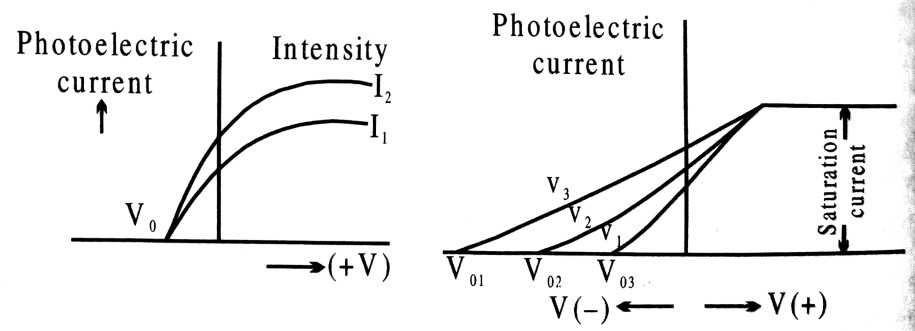Q.2. An electromagnetic wave of wave length λ is incident on a photosensitive surface of negligible work function. If the photo-electrons emitted from his surface have the de-Broglie wavelength λ1, prove that λ = (2mc/h)λ21.

Ans ⇒ From photoelectric equation, hv = φ0 + K.E. of electron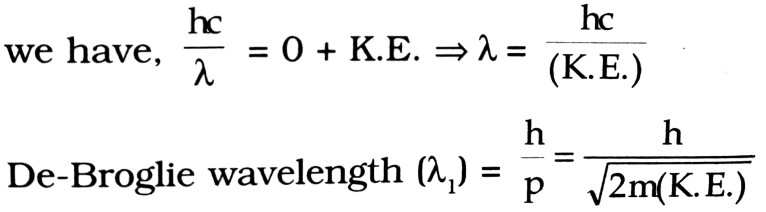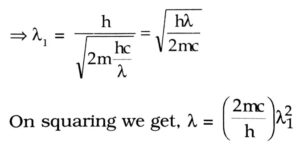Q.3. Define the terms threshold frequency di stopping potential in relation to the anomenon of photoelectric effect. How is the Fotoelectric current affected on increasing the Í frequency, (ii) intensity of the incident Fadiations and why ?

Ans ⇒ Threshold frequency : The minimum frequency of light which can free photo electron from a material is called the threshold frequency.

Stopping potential : It is that minimum negative notential given to anode in a photo cell for which the bhoto electric current become zero.

(i) Photo electric current remains unchanged as is does not depend upon the frequency of the incident light for the same intensity.

(ii) Since photo electric current is directly proportional to the intensity of incident radiation. On increasing intensity no. of photoelectrons also increase. So on increasing the intensity of incident radiation, photo-electric current also increases.

Q.4. Define the term, threshold frequency and work function in relation to photoelectric effects.

Ans ⇒ Threshold frequency : It is the minimum frequency of incident radiation required for photoelectric emission from a metal.

Work function: The amount of energy that must be supplied to an electron in order to just escape from the surface of a metal is called the work function of the metal.

Q.5. Show that the wavelength of electromagnetic radiation is equal to the de-Broglie wavelength of its quantum (photon).

Ans ⇒ For electromagnetic radiation, its velocity is given by
c= vλ
λ = c/v                                       …(i)
For photon, according to de-Broglie relation, the wavelength λ is given by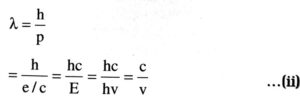From (i) and (ii), we find that wavelength e.m. radiation is equal to the de-Broglie wavelength of its quantum (photon).

Q.6. What is photoelectric effect ? Write Einstein’s photoelectric equation and use it to explain :
(i) Independence of maximum energy of emitted photo-electrons from the intensity of incident light, (ii) existence of a threshold frequency for emission of photoelectrons.

Ans ⇒ The ejection of electron from a metal surface by falling the radiation of suitable frequency on it is called photoelectric effect. The Einstein’s photoelectric equation is given as
Kmax = h(v–v0)

(i) The maximum kinetic energy of photoelectron is independent of intensity of incident radiation because is depends upon only the threshold frequency and frequency of incident radiation.

(ii) For photo emission v > v0, v < v0 there is on photo emission hence there exists a frequency below which photo emission is not possible.

Q.7. Draw a graph to show the variation of stopping potential with frequency of radiations incidents on a metal plate. How can the value of Plank’s constant be determined from this graph ?

Ans ⇒ Fig. showing the variation of stopping potential with variation of frequency of incident light.
From Einstein’s photoelectric equation the maximum K.E. of the photo-electron is given by where V0 is the stopping potential, v is the freqnuecy of incident radiation and W0 is the work function,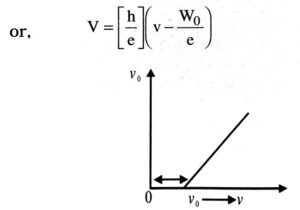This equation is the equation of a straight line (y = mx + c) with slope equal to h/e and intercept of y axis is equal to W0/e. Thus measuring the slope of the [V0 – v] graph and equating it to h/e, h can be calculated.

Q.8. Explain the laws of photoelectric emission on the basis of Einstein’s photoelectric equation. Write one feature of the photoelectric effect which cannot be explained on the basis of wave theory of light.

Ans ⇒ Einstein’s photoelectric equation is kmax = hv = φ, where kmax is maximum kinetic energy of photoelectron.
φ0 is work function
hv is energy of incident photon.
(i) As kmax depends linearly on V, its independent of intensity of radiation.
(ii) Since kmax must be non-negative
Above equation implies that photoelectric emission is possible only if
hv > φ0
or,              v > v0
where        v0 = φ0/h
It implies that there is a fundamental frequency below which no photoelectric emission is possible (whatever be the intensity).

(iii) Greater is the number of energy quanta available, greater is the number of electron absorbing the energy quanta. Thus, greater is the number of electron emitted. This explains why for v > v0 photoelectric current is proportional to intensity.

(iv) In Einsteins picture basic process is absorption of light quantum by an electron. This process is instantaneous. So, photoelectric emission is instantaneous.
Dependence of photoelectric current on intensity of light cannot be explained on the basis of wave theory of light.

Q.9. Obtain the expression for the maximum kinetic energy of the electrons emitted from a metal surface in terms of the frequency of the incident radiation and the threshold frequency,.

Ans ⇒ Consider a photon of light of frequency v, incident on a photosensitive metal surface. The energy of the photon (= hv) is spent in two ways.

(i) A part of energy is used in liberating electron from this metal sufrace which is equal to the work function W0 of the metal.

(ii) The rest of the energy of the photons is used in imparting the kinetic energy of the emitted photoelectron and mis its mass, then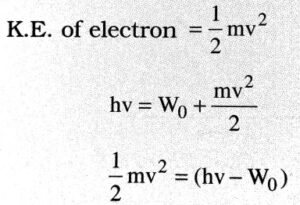The incident photon is of threshold frequency V0, then incident photon of energy hv0 is just sufficient to eject the electron, from the metal surface without imparting it any K.E. hence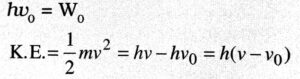As already established from Einstein’s photoelectric equation Max.
K.E. = hv – hv0                  …(i)

If V0 is the cut off potential and e is the charge on the electron, then Max.
K.E. = e V0                         … (ii)

If V0 is the threshold frequency, the work function
W0 = hv0                          … (iii)

From eqn. (i), (ii) and (iii), we have
eV0 = K.E.max = (hv – hv0) = h(v – v0)
It is the required relation between Max. K.E. threshold frequency and frequency of incident radiation.

Q.10. Why thermionie emission occurs in metals, why not in non-metals ?

Ans ⇒ In metals there are large number of free electrons while large amount of energy is needed to bring the electrons out from the non-metals, On coating of oxides of metals work function is reduced.

Q.11. Photo electric current is proportional to intensity of light while velocity of photo electrons depends upon frequency of light. Explain.

Ans ⇒ When light is made to incident on a metal plate then electrons are emitted which are called photoelectrons and phenomenon is called photoelectric effect. It was explained by Einstein on the basis of quantum theory of light. It is given by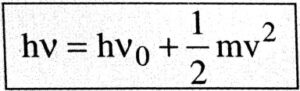, when hv is the energy of photon in which h is planck constant, v is frequency of photon, v0 is threshold frequency of photon and -mv is its kinetic energy in which m is the mas and u is the velocity of photon.
Higher the frequency of incident photon more will be the velocity of photo electrons. The number of electrons emitted from metal plate depends upon intensity of light (number of photon incident). So photon current depends upon number of photon i.e., intensity of light.

Q.12. Do X-rays and γ-rays have same nature and origin ?

Ans ⇒ X-rays and γ-rays are electromagnetic wave but the wavelength of γ-rays is 100 times smaller than wavelength of X-rays.
X-rays are produced, when a beam of fast moving electrons strike a solid target where as γ-rays are out come of the disintegration of radioactive elements like Uranium.

## Class 12th physics Subjective question in English

 S.N Physics Short Type Question English Medium 1. ELECTRIC CHARGES AND FIELDS 2. LECTROSTATIC POTENTIAL AND CAPACITANCE 3. CURRENT ELECTRICITY 4. MOVING CHARGES AND MAGNETISM 5. MAGNETISM AND MATTER 6. ELECTROMAGNETIC INDUCTION 7. ALTERNATING CURRENT 8. ELECTROMAGNETIC WAVES 9. RAY OPTICS AND OPTICAL INSTRUMENTS 10. WAVE OPTICS 11. DUAL NATURE OF MATTER AND RADIATION 12. ATOMS 13. NUCLEI 14. SEMI CONDUCTOR ELECTRONICS 15. COMMUNICATION SYSTEMS